## 20150421

### Pitch Axis Considered Harmful

Musical Inversion is not a reflection, it's a subtraction. You may hear about a 'pitch axis' when talking about inversion. This is to justify the (mistaken) idea that – to get a musical (phrase) inversion – you reflect the notes of the phrase along a horizontal line, the so-called pitch axis.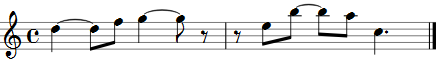and ‘reflect’ it about a supposed pitch axis, let’s pick the D of the first note. We get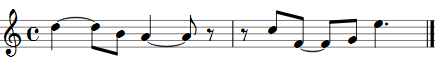As it happens, all the notes are in the same key as the original (C major or A minor). The ‘essence’ of the phrase is preserved.

But suppose instead we had reflected that first phrase along the middle line of the stave, i.e. suppose the pitch axis to be the B. We’d get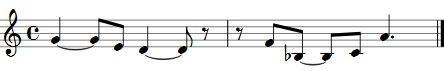There’s a B♭ in the second bar because where the original rose a perfect fifth (i.e. seven semitones) from E to B, our inversion must perforce fall a perfect fifth from F to (the out-of-scale) B♭. We’ve broken something - we’ve lost our C-majority. But let’s not worry about it. Fix (or kludge) it by going to the nearest in-scale note (a B). Or maybe we’ve changed key. Perhaps to F major? A musician isn’t all that bothered.

In fact, in this particular case, the ‘pitch axis’ of D is the only one that works, to the extent that it keeps you in the original scale. I.e. Inversion may be a scale preserving operation, but in general (in actuality, 6/7ths of the time, i.e. mostly) it’s not.

Clearly, if you want to stay in C major when you invert then your starting note must be one of seven C, D, E, F, G, A or B. Mutatis – of course – mutandis for other keys.

Starting on the original D (where the pitch axis is the very line you’re already on), or the F above it (where the pitch axis would be the E gap between them), or the B below it (where the pitch axis is the C between them), or the G below (where our first mid-line pitch axis of B was) the first D etc all seem reasonable.

But starting on the C below the D? That would mean the pitch axis is a C#. We know that C# exists - but shouldn’t it bother us just a bit that we must allow a pitch axis to be on a note not even in the scale we’re trying to preserve? Well, OK, let it go. After all, nobody said a pitch axis must be a note in the scale.

So let’s proceed on that basis. As it happens, an inversion starting on C would take us down a minor third to A (reflecting the first minor third transition from D up to F), down again a tone to G (reflecting the original’s tone up from F to G), to our two quaver rest, then up a minor third (reflecting the minor third drop from G to E) to – oops, again a B♭.

But hang on a minute. Before we go any further, once we’ve allowed that a C# exists then there’s another problem. Starting on the B below the D meant that we were assuming a pitch axis of C. But in actual fact the pitch axis should surely be (since there are three semitones between that B and D) one and a half semitones up from B, i.e. a quarter-tone lying between C and C#. It’s not even in the chromatic scale we grudgingly allowed a pitch axis to inhabit!

This is getting silly. The idea of pitch axis has been stretched beyond reason. It’s broken. Throw it away. It just doesn’t work.

So what, if anything, does work?

Subtraction does. Always, and reliably and consistently. But you have to know your modes and your moods. To see this, we consider an abstraction of a major scale – in any key whatsoever – lying embedded within a standard western twelve tone musical octave.

Any major scale is represented – in modulo 12 arithmetic – by the seven note sequence of integers 0, 2, 4, 5, 7, 9, 11. Corresponding, for example, to the notes C, D, E, F, G, A, B of the C major scale. Or to the notes E, F#, G#, A, B, C#, D of the E major scale, etc.

The 0 is the tonic note, the 2 represents the two semitones above it supertonic, the 4 the mediant (to continue to use these old musical terminologies) two whole tones or four semitones above the tonic (aka the major third), the 5 the five-semitonally distant subdominant (or fourth), the 7 the dominant (aka the fifth). the 9 the submediant and the 11 the subtonic last semitone below the octave.

So any musical phrase within any chosen major scale may be written (in pitch terms alone, we’re not concerned with duration, or with rests) as a sequence of 0s, 2s, 4s, 5s, 7s, 9s and 11s. Note that if you do wish to consider the octaves important just add (or subtract) the relevant 12 – e.g. 14 is still a ‘2’, –13 is still an ‘11’, etc (it’s all just ‘clock arithmetic’). If you’re bothered that chords aren’t covered here, that’s just a notational problem – write the chord sequence C, Em, Dm7 as [0,4,7] [4,7,11] [2,5,9,12 (or 0)] if you like, or come up with your own scheme – it really doesn’t affect anything discussed here.

Inversion as subtraction, not reflection, simply means that you need a note – represented by one of the seven values in your scale – to subtract your sequence from.

For the major scale, the subtraction happens to be from 4 (or – when convenient, after adding 12 - 16). I.e subtracting the seven values 0, 2, 4, 5, 7, 9, 11 respectively from 4 exactly reproduces the original scale – but in a different order (viz. 4, 2, 0, 11, 9, 7, 5).

4 – 0 = 4; 4 – 2 = 2; 4 – 4 = 0; 16 – 5 = 11; 16 – 7 = 9; 16 – 9 = 7; 16 – 11 = 5

That’s the only number (modulo 12) that works with the major scale. Try any other, for example 7, and you get 7–0=7 (ok); 7-2=5 (ok); 7-4=3 (not ok!); 7-5=2 (ok); 7-7=0 (ok); 7-9=-2 (equivalent to 19-9=10) (not ok!); 7-11=-4 (equivalent to 19-11=8) (not ok). I.e. subtracting from 7 pulls in the out-of-scale number 10, 8 and 3 (i.e. B♭, A♭ and E♭ in C major) - we've upset the applecart even as far as turning something written in a major key into its own minor. That's a pretty significant change there!.

So our first trial inversion worked – not because we happened to accidentally pick the correct pitch axis of D – but because we happened to subtract each note of the original phrase from the mediant of the major scale.

What about the minor scale? This is represented by the seven member set (0, 2, 3, 5, 7, 8, 10). If you want to invert in the minor scale, you subtract from its subtonic. Again, it’s the only one that works, keeping you in your minor scale. For example, to invert an A minor phrase, you subtract each note in the phrase from its subtonic G.

Using our first example phrase considered as one in A minor rather than C major, the sequence D, F, G, E, B, A, C is represented by 5, 8, 10, 7, 2, 0, 3. Subtracting each of those numbers from 10 gives you, respectively, 5, 2, 0, 3, 8, 10, 7 – i.e. all of them within the (abstraction of the) A minor scale.

As with 4 for the major scale, 10 is the only one which works. Subtracting from any of the other six possible numbers will introduce a number (modulo 12) which lies outside the minor scale. E.g. 2–3 brings in a –1, i.e. an 11, which is a ‘wrong note’. As does 4–5, or 5–8 = –3, aka 9 – wrong again. Or 7–8, another –1; or 8–2 = 6 (nope, missing in the minor scale).

So to invert in the major, subtract from its mediant (or its major third, if you will). To invert in the minor, subtract from its subtonic - its (minor) seventh.

But wait. Another (but other worldly) name for the major scale is the Ionian Mode. Another name for the minor scale is the Aeolian Mode. These are two of the seven ‘ancient’ modes corresponding to the seven notes of a diatonic scale, considered as starting on the ‘wrong’ (for six of them, anyway) note. Each mode has its own distinct subtraction point from which mode/scale preserving inversions can be made. Here’s the list

• Ionian (0,2,4,5,7,9,11) inverts from its mediant (4)
• Dorian (0,2,3,5,7,9,10) inverts from its tonic (0)
• Phrygian (0,1,3,5,7,8,10) inverts from its submediant (8)
• Lydian (0,2,4,6,7,9,11) inverts from its subdominant (6)
• Mixolydian (0,2,4,5,7,9,10) inverts from its supertonic (2)
• Aeolian (0,2,3,5,7,8,10) inverts from its subtonic (10)
• Locrian (0,1,3,5,6,8,10) inverts from its dominant (6)

ionian
(major)
dorian phrygian lydian mixolydian aeolian
(minor)
locrian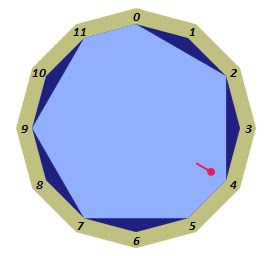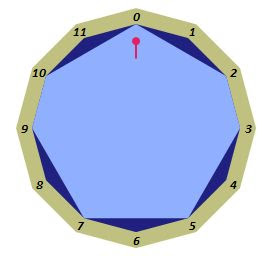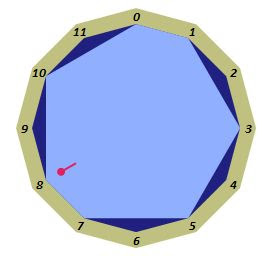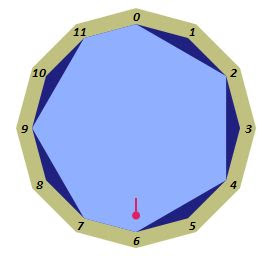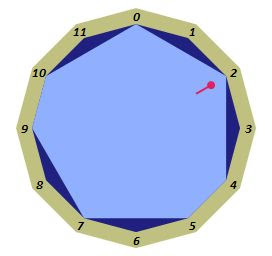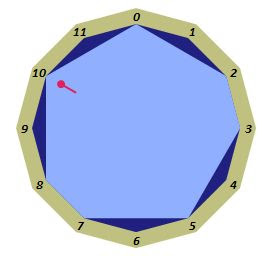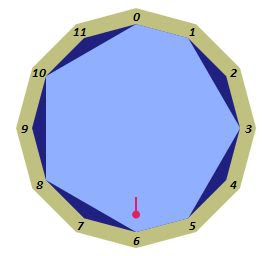Seven modal scales as rotations of a heptagon

Again, we emphasise that the modal numbers that you subtract from (add as many 12s as you require) are the only ones – out of the seven possibilities for each – which will preserve the mode. The ‘pitch axis’ – or reflection – idea is quite unnecessary. We'll see more of these polygons in the next article.

Inversion as subtraction carries over into other scales too. For example, Jazz musicians are rather partial to the octatonic (aka diminished) scale which – as its name suggests, is an eight note scale (but still embedded within the chromatic 12-tone universe). Its alternating wholetone/semitone stepping nature can be represented as the eight note set 0, 2, 3, 5, 6, 8, 9, 11 – or alternatively 0, 1, 3, 4, 6, 7, 9, 10. To invert a phrase written in this scale, you have a bit more freedom than that which the traditional heptatonic modes permit you. You may subtract from any one of four of its scale notes, i.e. from 2 or 5 or 8 or 11 (or 1, 4, 7, 10 in the alternate version). The term tonic may still apply to such a scale, but the notions of dominant, supertonic etc won’t fit any more – we’re one short.

octatonic diminished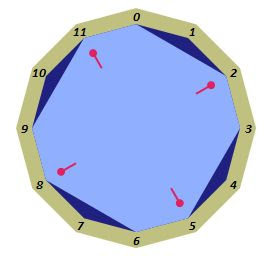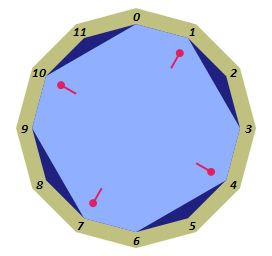Octatonic Scales with quadruple inversion points

And pentatonic scales also have inversions. The (five note) major pentatonic scale is represented by 0, 2, 4, 7, 9. To invert a phrase in this scale, subtract its notes from 4 (just like the ordinary major scale). 4-0=4, 4-2=2, 4-4=0, 4(=16)-7=9, 4(=16)-9=7. Subtraction from the other notes (0, 2, 7 or 9) won’t work. The minor pentatonic – i.e. 0, 3, 5, 7, 10 – like the ordinary 7 note minor scale – is invertible from 10. I.e. 10-0=10, 10-3=7, 10-5=5, 10-7=3, 10-10=0. Like the ordinary heptatonic/diatonic/modal scales, these inversion points are unique.

And distinct – corresponding to the seven modes of the diatonic scales are the five ‘modes’ of pentatonic ones. Being unaware of any formal names for these, we’ll just go by analogy:

• io-pentatonic (aka major) (0,2,4,7,9) inverts from (4)
• do-pentatonic (0,2,5,7,10) inverts from (0)
• ph-pentatonic (0,3,5,8,10) inverts from (8)
• mi-pentatonic (0,2,5,7,9) inverts from (2)
• ae-pentatonic (aka minor) (0,3,5,7,10) inverts from (10)

io
(major)
do ph mi ae
(minor)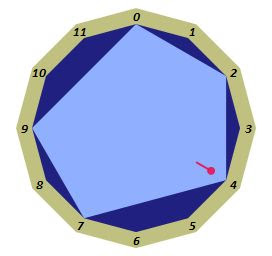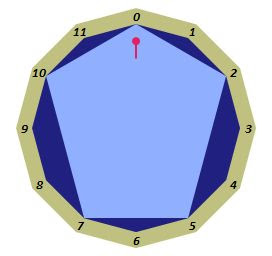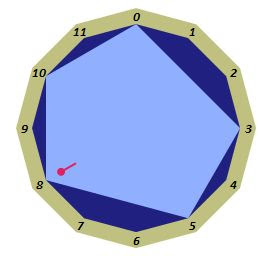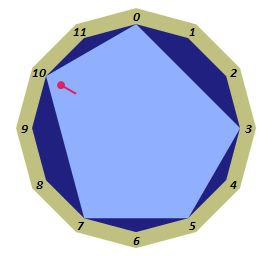Five Invertible Pentatonic Scales as rotated pentagons

These are just a few of the scales which can be fashioned from the 12 notes available to us. One may consider scales consisting of all 12 notes – there’s only the one, the full chromatic scale. Inversion in that scale is of course possible from any of its notes since subtraction from anything is bound to produce one of the 12 notes you have. Scales of 11 notes – of which there are of course 12 actual (each with one of the 12 notes missing) – but only one in practice (they’re all just transposed versions of each other, i.e. all represented by the same set 0,1,2,3,4,5,6,7,8,9,10) must have limited inversion (you will be unable to subtract from 11, because 11-0=11, which isn’t in the scale). Symmetrical with 11 note scales are the 12 actual (but only one really) single note scales which can – pretty clearly – invert only by subtraction from their only note – ‘One note Samba’ considered trivial. There’s also only the one zero-note scale. but the only piece written in that may be Cage’s 4’33” assuming it’s written in any scale at all.

There are – technically – 60 scales comprising 10 notes (and, symmetrically, 2 notes) but – again in practice – many of them are merely transpositions of each other and there are really only six distinct patterns of 10 (or 2) note scales (each may be considered to have 10 – or 2 - ‘modes’ starting on different notes).

Of the 171 possible 9 note scales there are 19 distinct patterns – each with something akin to 9 modes, and this is also (symmetrically) the case for the 19 possible patterns for 3 note scales (with each of their 3 ‘modes’).

The ‘jazz octatonic’ isn’t the only way to arrange an 8 note scale. There are 43 different patterns available. Likewise 4 note scales.

There are in fact 66 different ways to have 7 note scales. The two (or seven) we are familiar with represent just one of these 66. Being - as they are - only transpositions of each other in their various modes. Most of them are rather pathological. For example a run of seven consecutive semitones might not provide a very interesting scale. Similarly there are 66 pentatonic patterns.

Finally there are 80 distinct hexatonic scale patterns, only one of them being the - possibly familiar – whole tone scale. The whole tone scale in particular is – like the full 12 tone scale – invertible from any of its 6 constituent scale notes.

By no means do all of these (350) scales permit in-scale-phrasal-inversion. And in those that do, reflection – or pitch axis – is of no real use in any of them except by accident.

Here’s a table of all possible N note scales, and how many of them are properly invertible

#Notes #Scales #Invertible
12 1 1
11 1 1
10 6 6
9 19 5
8 43 15
7 66 10
6 80 20
5 66 10
4 43 15
3 19 5
2 6 6
1 1 1

That there are exactly as many, say, 4 note scales as there are 8 note scales should be reasonably obvious. A scale with N notes missing from it can be considered the anti-scale of the corresponding N note one and although musically it will sound rather different (except possibly when N is 6), there’ll be exactly one such scale to correspond to its N note scale. We’ve omitted the no-note scale (the anti-12-tone) as being too musically challenging (Cage again).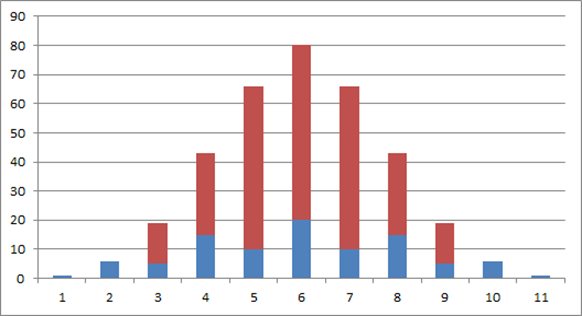Diagram showing that invertible scales (blue) are in the minority